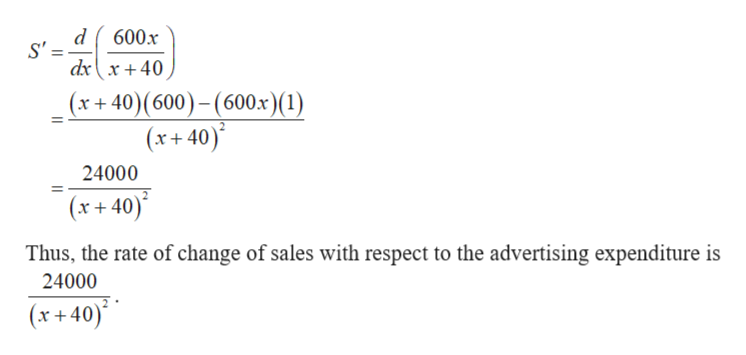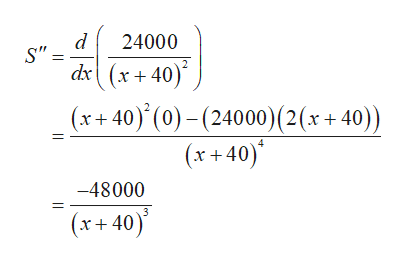The sales of a product S(in thousands of dollars) are given byS= 600x/x+40where x is the advertising expenditure(in thousands of dollars)(a) Find the rate of change of sales with respect to advertising expenditure.(b) Use the second derivative to find how this rate is changing at x=20.(c) Interpret your result in part (b).

Question

The sales of a product S(in thousands of dollars) are given by

S= 600x/x+40

where x is the advertising expenditure(in thousands of dollars)

(a) Find the rate of change of sales with respect to advertising expenditure.

(b) Use the second derivative to find how this rate is changing at x=20.

(c) Interpret your result in part (b).

Step 1

Given:

The function that repesents the sales of a product S is

Step 2

a)

Obtain the derivative of the given function with respect to x.help_outlineImage Transcriptionclose600x dx{x+ 40 (x+ 40)(600)-(600x) (1) (x+40) 24000 (x+40) Thus, the rate of change of sales with respect to the advertising expenditure is 24000 (x+40) fullscreen
Step 3

b)

Derivate the above obtained S’ w...help_outlineImage Transcriptionclosed S" dx(x+40) 24000 (x40) (0)(24000) (2 (x + 40)) (x40) -48000 (x+40) fullscreen

Want to see the full answer?

See Solution

Want to see this answer and more?

Our solutions are written by experts, many with advanced degrees, and available 24/7

See Solution
Tagged in

Other